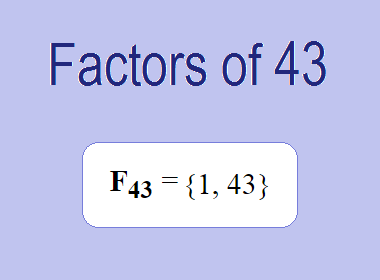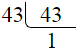# Factors of 43The factors of 43 are 1 and 43 i.e. F43 = {1, 43}. The factors of 43 are all the numbers that can divide 43 without leaving a remainder. 43 is a prime number, so it is divisible by 1 and 43 only.

We can check if these numbers are factors of 43 by dividing 43 by each of them. If the result is a whole number, then the number is a factor of 43. Let's do this for each of the numbers listed above:

·        1 is a factor of 43 because 43 divided by 1 is 43.

·        43 is a factor of 43 because 43 divided by 43 is 1.

## Properties of the Factors of 43

The factors of 43 have some interesting properties. One of the properties is that the sum of the factors of 43 is equal to 44. We can see this by adding all the factors of 43 together:

1 + 43 = 44

Another property of the factors of 43 is that the only prime factor of 43 is 43 itself.

********************

********************

## Applications of the Factors of 43

The factors of 43 have several applications in mathematics. One of the applications is in finding the highest common factor (HCF) of two or more numbers. The HCF is the largest factor that two or more numbers have in common. For example, to find the HCF of 43 and 86, we need to find the factors of both numbers and identify the largest factor they have in common. The factors of 43 are 1, and 43. The factors of 86 are 1, 2, 43, and 86. The largest factor that they have in common is 43. Therefore, the HCF of 43 and 86 is 43.

Another application of the factors of 43 is in prime factorization. Prime factorization is the process of expressing a number as the product of its prime factors. The prime factor of 43 is 43 since it is only the prime number that can divide 43 without leaving a remainder. Therefore, we can express 43 as:

43 = 43

We can do prime factorization by division method as given below,43 = 43

Since 43 is a prime number, there is no factor tree of 43.

## Conclusion

The factors of 43 are the numbers that can divide 43 without leaving a remainder. The factors of 43 are 1, and 43. The factors of 43 have some interesting properties, such as having a sum of 44. The factors of 43 have several applications in mathematics, such as finding the highest common factor and prime factorization.